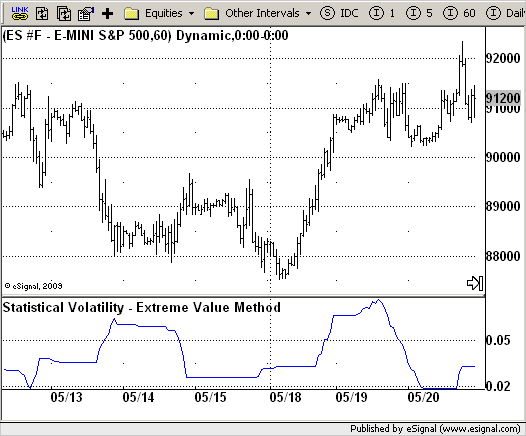# Statistical Volatility - Extreme Value Method

ICE Data Services -

 VolatilityExtVal.efs EFSLibrary - Discussion Board

File Name: VolatilityExtVal.efs

Description:
Statistical Volatility - Extreme Value Method

Formula Parameters:
nLength : 30

Notes:
This indicator used to calculate the statistical volatility, sometime
called historical volatility, based on the Extreme Value Method.`/*********************************Provided By: eSignal (Copyright c eSignal), a division of Interactive Data Corporation. 2009. All rights reserved. This sample eSignal Formula Script (EFS) is for educational purposes only and may be modified and saved under a new file name. eSignal is not responsible for the functionality once modified. eSignal reserves the right to modify and overwrite this EFS file with each new release.Description: Statistical Volatility - Extreme Value Method Version: 1.0 05/22/2009 Formula Parameters: Default: nLength 30 Notes: This indicator used to calculate the statistical volatility, sometime called historical volatility, based on the Extreme Value Method. Please use this link to get more information about Volatility. **********************************/var fpArray = new Array();var bInit = false;function preMain() { setStudyTitle("Statistical Volatility - Extreme Value Method"); setCursorLabelName("Xtrme Vlty"); var x = 0; fpArray[x] = new FunctionParameter("nLength", FunctionParameter.NUMBER); with(fpArray[x++]) { setLowerLimit(1); setDefault(30); } }var xVolatilityExtVal = null;function main(nLength) {var nBarState = getBarState();var nVolatilityExtVal = 0; if (nBarState == BARSTATE_ALLBARS) { if (nLength == null) nLength = 30; } if (bInit == false) { xVolatilityExtVal = efsInternal("Calc_VolatilityExtVal", nLength); bInit = true; } nVolatilityExtVal = xVolatilityExtVal.getValue(0); if (nVolatilityExtVal == null) return; return nVolatilityExtVal;}var bSecondInit = false;var xClose = null;var xMaxC = null;var xMinC = null;var xMaxH = null;var xMinL = null;function Calc_VolatilityExtVal(nLength) {var nRes = 0;var i = 0;var SqrTime = Math.sqrt(253 / nLength); if (bSecondInit == false) { xClose = close(); xMaxC = upperDonchian(nLength, xClose); xMinC = lowerDonchian(nLength, xClose); xMaxH = upperDonchian(nLength); xMinL = lowerDonchian(nLength); bSecondInit = true; } if (xMaxC.getValue(0) == null) return; nRes = ((0.6 * Math.log(xMaxC.getValue(0) / xMinC.getValue(0)) * SqrTime) + (0.6 * Math.log(xMaxH.getValue(0) / xMinL.getValue(0)) * SqrTime)) * 0.5; if (nRes < 0) nRes = 0.0; if (nRes > 2.99) nRes = 2.99; return nRes;}`